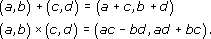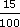Look at the shapes below. The symmetry of the shape on the left and its relationship to the shape on the right can be thought of in two ways:

• Fold the left-hand shape along the central line. Then one side lies exactly on top of the other, and gives the shape on the right.

• Imagine a mirror placed along the central dotted line. The reflection in the mirror gives the other half of the shape.

Author(s): The Open University

All circles are the same shape – they can only have different sizes.

In a circle, all the points are the same distance from a point called the centre. The centre is often labelled with the letter O.Author(s): The Open UniversityA quadrilateral is a shape with four straight sides.
Author(s): The Open University

When two straight lines cross, they form four angles. In the diagram below, these angles are labelled α, β, θ and φ and referred to as alpha, beta, theta and phi. The angles opposite each other are equal. They are called vertically opposite angles. Here α and β are a pair of vertically opposite angles, as are θ and φ. Although such angles are called ‘vertically opposite’, they do not need to be vertically above and bel
Author(s): The Open University

## Question 1

A company carried out a survey, recording how staff in a particular office spent their working time. The table shows the average number of minutes spent in each hour on various activities.

Author(s): The Open University

You can use the fact that the sum of angles at a point is 360° to draw a pie chart.

## Example 4

Over a five-year period a mathematics tutor found that 16 of her students gained distinctions, 32 gained pass grades and 12 failed to complete the course. Draw a pie chart to re
Author(s): The Open University

## SAQ 26

Find the distance between the numbers 2 − i and 1 + 3i.

Author(s): The Open University

In this section we have seen that the complex number system is the set R × R together with the operations + and × defined byFrom this, one can justify the performance of ordinary algebraic operations on expressions of the form
Author(s): The Open University

Section 6 contains solutions to the exercises that appear throughout sections 1-5.

Click the link below to open the solutions (13 pages, 232KB).

Section 6

Author(s): The Open University

In Section 5 we show how functions may be used to sketch curves in the plane, even when these curves are not necessarily the graphs of functions.

Click the link below to open Section 5 (8 pages, 151KB).

Section 5

Author(s): The Open University## Study another free course

There are more than 800 courses on OpenLearn for you t
Author(s): The Open University

Discount can be calculated in the same way as an increase by a percentage. For example, £8 with 15% discount means you actually pay

£8 less (15% of £8)

15% of 8 =× 8 =
Author(s): The Open University

## Activity 20

Convert each of the following to percentages. Round off the percentages to whole numbers.

• (a)

• (i) 0.8

• (ii) 0.
Author(s): The Open University

## Activity 17

Express each of the following percentages as fractions:

• (a) 40%

• (b) 8%

• (c) 70%

• (d)
Author(s): The Open University

## Activity 14

A piece of computer software is to be developed by a team of programmers. It is estimated that a team of four people would take a year. Which of the following times is the length of time taken by three program
Author(s): The Open University

## Activity 5

Convert each of the following fraction ratios to decimal ratios.

• (a) [Image_Link]https://www.open.edu/op
Author(s):
The Open University

Although ratios are often given as fractions, they can also be expressed as decimals. You need to deal with a mixture of fractions and decimals, and to compare ratios given in either form, so you need to be able to convert between the two forms.

## Example 4

The ratio of the circ
Author(s): The Open University

Time conversions are also ratios. The ratio of time measured in minutes to time measured in seconds is one to sixty (1:60), as there are sixty seconds in a minute.

## Example 2

Adam's grandfather ran a mile in
Author(s): The Open University

To make short crust pastry, one recipe book says ‘use one part of fat to two parts of flour’; another recipe says ‘use fat and flour in the ratio of one to two’; and yet another says ‘use half as much fat as flour’. These are different ways of expressing the same ratio. Ratios are often expressed as fractions. So in this case:

[Image_Link]https://www.open.edu/openlearn/ocw/pluginfile.php/
Author(s): The Open University

The content acknowledged below is Proprietary (see and conditions made available under a Creative Commons Attribution-NonCommercial-ShareAlike 4.0 Licence) and used under licence.

Course image: rod
Author(s): The Open University